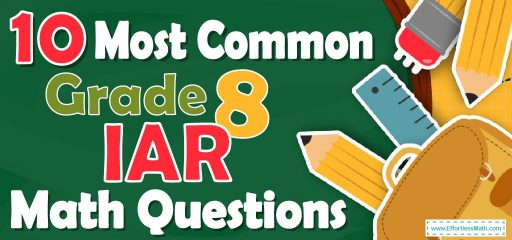# 10 Most Common 8th Grade IAR Math QuestionsIs passing the 8th-Grade IAR math a necessity for you? So you can improve your math skills for the 8th-Grade IAR test by using the 10 Most Common 8th-Grade IAR Math Questions!

The math section of the 8th-Grade IAR is always a challenging section for 8th-grade students. but do not worry! Familiarity with the common questions of the 8th Grade IAR Math test will fully prepare you for this section.

In this article, we try to help 8th-grade students gain a better understanding of 8th Grade IAR Math test questions by providing 10 Most Common 8th Grade IAR Math Questions.

Using these Most Common 8th Grade IAR Math Questions will help 8th-grade students review their math knowledge and know which areas of math they have to complete their information.

Make sure to follow some of the related links at the bottom of this post to get a better idea of what kind of mathematics questions students need to practice.

The Absolute Best Book to Ace 8th Grade IAR Math Test

## 10 Sample 8th Grade IAR Math Practice Questions

1- A chemical solution contains $$4\%$$ alcohol. If there is $$24\:\ ml$$ of alcohol, what is the volume of the solution?

A. $$240\:\ ml$$

B. $$480\:\ ml$$

C. $$600\:\ ml$$

D. $$1200\:\ ml$$

2- What is the area of the shaded region?

A. $$31$$

B. $$40$$

C. $$64$$

D. $$80$$

3- A $$40$$ shirt now selling for $$28$$ is discounted by what percent?

A. $$20 \%$$

B. $$30 \%$$

C. $$40 \%$$

D. $$60 \%$$

4- How much interest is earned on a principal of $$5000$$ invested at an interest rate of $$5\%$$ for four years?

A. $$250$$

B. $$500$$

C. $$1000$$

D. $$2000$$

5- A swimming pool holds $$2,000$$ cubic feet of water. The swimming pool is $$25$$ feet long and $$10$$ feet wide. How deep is the swimming pool?

6- The price of a car was $$20,000$$ in $$2014$$, $$16,000$$ in $$2015$$ and $$12,800$$ in $$2016$$. What is the rate of depreciation of the price of a car per year?

A. $$15 \%$$

B. $$20 \%$$

C. $$25 \%$$

D. $$30 \%$$

7- What is the area of the shaded region if the diameter of the bigger circle is $$12$$ inches and the diameter of the smaller circle is $$8$$ inches?

A. $$16 π$$

B. $$20 π$$

C. $$36 π$$

D. $$80 π$$

8- What is the area of an isosceles right triangle that has one leg that measures $$6 cm$$? _________

9- A taxi driver earns $$9$$ per $$1$$-hour work. If he works $$10$$ hours a day and in $$1$$ hour he uses $$2$$-liters petrol with price $$1$$ for $$1$$-liter. How much money does he earn in one day?

A. $$90$$

B. $$88$$

C. $$70$$

D. $$60$$

10- What is the solution to the following system of equations?
$$\frac{-x}{2}+ \frac{y}{4} = 1$$
$$\frac{-5y}{6}+ 2x = 4$$

A. $$x=48,y=22$$

B. $$x=50,y=20$$

C. $$x=20,y=50$$

D. $$x=22,y=48$$

Best 8th Grade IAR Math Prep Resource

1- C
$$4\%$$ of the volume of the solution is alcohol. Let $$x$$ be the volume of the solution.
Then: $$4\%$$ of $$x = 24 ml ⇒ 0.04 x = 24 ⇒ x = 24 ÷ 0.04 = 600$$

2- B
Use the area of the rectangle formula $$(s = a × b)$$.
To find the area of the shaded region subtracts the smaller rectangle from the bigger rectangle.
$$S_{1} – S_{2} = (10 ft × 8ft) – (5ft × 8ft) ⇒ S_{1} – S_{2} = 40ft$$

3- B
Use the formula for Percent of Change
$$\frac{New \space Value-Old \space Value}{Old \space Value}× 100 \%$$
$$\frac{28-40}{40}× 100 \% = –30 \%$$(negative sign here means that the new price is less than old price).

4- C
Use simple interest formula:
$$I=prt$$
(I = interest, p = principal, r = rate, t = time)
$$I=(5000)(0.05)(4)=1000$$

5- 8
Use the formula of rectangle prism volume.
$$V = (length) (width) (height) ⇒ 2000 = (25) (10) (height) ⇒ height = 2000 ÷ 250 = 8$$

6- B
Use this formula: Percent of Change
$$\frac{New \space Value-Old \space Value}{Old \space Value}× 100 %$$
$$\frac{16000-20000}{20000}× 100 %$$
$$= 20 \% \space and \space \frac{12800-16000}{16000}× 100 \% = 20 \%$$

7- B
To find the area of the shaded region subtract the smaller circle from the bigger circle.
$$S_{bigger}-S_{smaller} =π(r_{bigger})^2 -π(r_{smaller})^2⇒S_{bigger}-S_{smaller}=π(6)^2-π(4)^2$$
$$⇒ 36 π – 16π = 20 π$$

8- 18
$$a = 6 ⇒$$ area of the triangle is:
$$\frac{1}{2}(6×6)=\frac{36}{2}=18 \space cm^2$$

9- C
$$9×10=90$$
Petrol use: $$10×2=20 \space liters$$
Petrol cost: $$20×1=20$$
Money earned: $$90-20=70$$

10- D
$$\frac{-x}{2}+ \frac{y}{4} = 1$$
$$\frac{-5y}{6}+ 2x = 4$$
Multiply the top equation by $$4$$. Then,
$$-2x+ y = 4$$
$$\frac{-5y}{6}+ 2x = 4$$
$$\frac{1}{6}y=8→y=48$$, plug in the value of y into the first equation →$$x=22$$

The Best Books to Ace 8th Grade IAR Math Test

### What people say about "10 Most Common 8th Grade IAR Math Questions - Effortless Math: We Help Students Learn to LOVE Mathematics"?

No one replied yet.

X
27% OFF

Limited time only!

Save Over 27%

SAVE $5 It was$18.99 now it is \$13.99